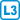## Series with binomial coefficients

• #### Variant

$$\sum\limits_{k=0}^n k\binom{n}{k}$$

• #### Variant

$$\sum\limits_{k=0}^n k^2\binom{n}{k}$$

• #### Variant

$$\sum\limits_{k=0}^{2n} (-1)^k\binom{4n}{2k}$$

• #### Variant

$$\sum\limits_{k=0}^{2n-1} (-1)^k\binom{4n}{2k+1}$$